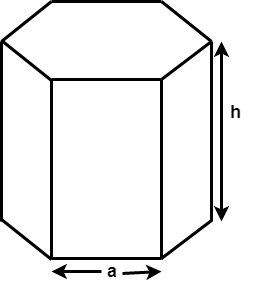GeeksforGeeks App
Open AppBrowser
Continue

# Surface Area and Volume of Hexagonal Prism

Given a Base edge and Height of the Hexagonal prism, the task is to find the Surface Area and the Volume of hexagonal Prism. In mathematics, a hexagonal prism is a three-dimensional solid shape which have 8 faces, 18 edges, and 12 vertices. The two faces at either ends are hexagons, and the rest of the faces of the hexagonal prism are rectangular.where a is the base length and h is the height of the hexagonal prism.

Surface Area =Volume =Examples:

Input : a = 4, h = 3
Output : Surface Area: 155.138443
Volume: 124.707657

Input : a = 5, h = 10
Output : Surface Area: 429.904
Volume: 649.519

## C++

 // C++ program to find the Surface Area// and Volume of Hexagonal Prism. #include using namespace std; // Function to calculate Surface areavoid findSurfaceArea(float a, float h){    float Area;     // Formula to calculate surface area    Area = 6 * a * h + 3 * sqrt(3) * a * a;     // Display surface area    cout << "Surface Area: " << Area;    cout << "\n";} // Function to calculate Volumevoid findVolume(float a, float h){    float Volume;     // formula to calculate Volume    Volume = 3 * sqrt(3) * a * a * h / 2;     // Display Volume    cout << "Volume: " << Volume;} // Driver Codeint main(){    float a = 5, h = 10;         // surface area function call    findSurfaceArea(a, h);     // volume function call    findVolume(a, h);     return 0;}

## Java

 // Java program to find the Surface Area// and Volume of Hexagonal Prism. import java.io.*; class GFG {            // Function to calculate Surface area    static void findSurfaceArea(float a, float h)    {        float Area;         // Formula to calculate surface area        Area = 6 * a * h + 3 * (float)(Math.sqrt(3)) * a * a;             // Display surface area        System.out.println("Surface Area: " + Area);    }         // Function to calculate Volume    static void findVolume(float a, float h)    {        float Volume;             // formula to calculate Volume        Volume = 3 * (float)(Math.sqrt(3)) * a * a * h / 2;             // Display Volume        System.out.println("Volume: " + Volume);    }     // Driver code    public static void main (String[] args)    {        float a = 5, h = 10;             // surface area function call        findSurfaceArea(a, h);             // volume function call        findVolume(a, h);    }}

## Python3

 # Python3 program to find the# Surface Area and Volume# of Hexagonal Prism.import math # Function to calculate# Surface areadef findSurfaceArea(a, h):    Area = 0;     # Formula to calculate    # surface area    Area = (6 * a * h +            3 * math.sqrt(3) * a * a);     # Display surface area    print("Surface Area:",          round(Area, 3));     # Function to# calculate Volumedef findVolume(a, h):    Volume = 0;     # formula to    # calculate Volume    Volume = (3 * math.sqrt(3) *                a * a * h / 2);     # Display Volume    print("Volume:",           round(Volume, 3)); # Driver Codea = 5;h = 10; # surface area# function callfindSurfaceArea(a, h); # volume function callfindVolume(a, h); # This code is contributed# by mits

## C#

 // C# program to find the// Surface Area and Volume// of Hexagonal Prism.using System; class GFG{             // Function to calculate    // Surface area    static void findSurfaceArea(float a,                                float h)    {        float Area;         // Formula to calculate        // surface area        Area = 6 * a * h + 3 *               (float)(Math.Sqrt(3)) * a * a;             // Display surface area        Console.WriteLine("Surface Area: " +                                      Area);    }         // Function to    // calculate Volume    static void findVolume(float a,                           float h)    {        float Volume;             // formula to calculate Volume        Volume = 3 * (float)(Math.Sqrt(3)) *                              a * a * h / 2;             // Display Volume        Console.WriteLine("Volume: " +                              Volume);    }     // Driver code    public static void Main ()    {        float a = 5, h = 10;             // surface area        // function call        findSurfaceArea(a, h);             // volume function call        findVolume(a, h);    }} // This code is contributed// by anuj_67.

## PHP

 

## Javascript

 

Time complexity : O(1)  as performing constant operations
Auxiliary Space : O(1)

My Personal Notes arrow_drop_up